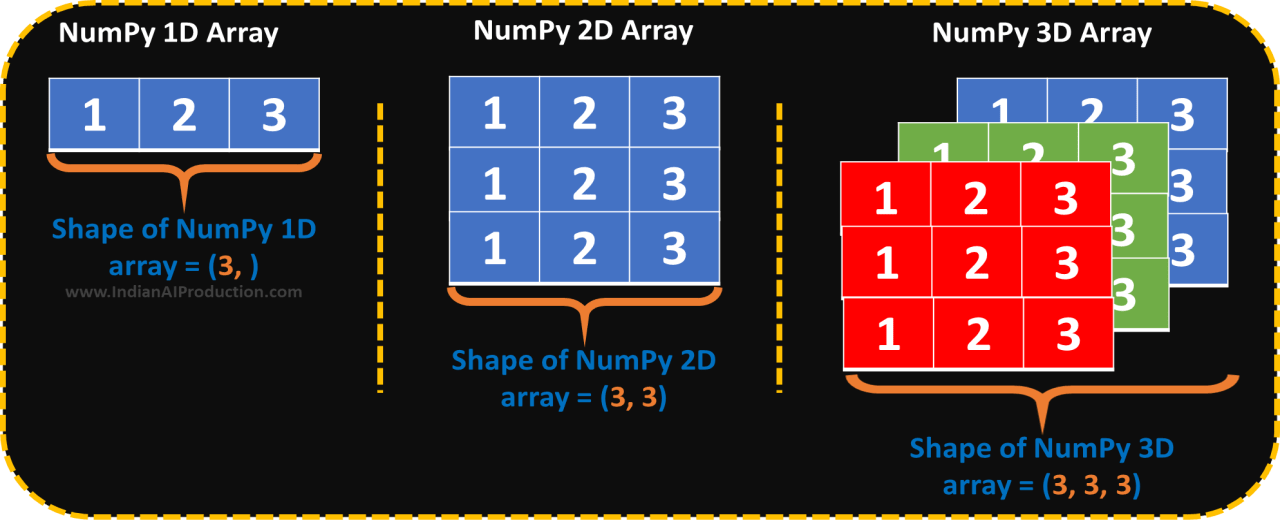# Python NumPy shape – Python NumPy Tutorial

## Python NumPy array shape Function

The NumPy shape function helps to find the number of rows and columns of python NumPy array. The numpy.shape() function gives output in form of tuple (rows_no, columns_no).

Syntax: np.shape(array)### The shape of a Numpy 1D array

```import numpy as np # import numpy package
arr_1D = np.array([1, 2, 3]) # create 1D array
print("One dimentional NumPy array : \n", arr_1D) # print arr_1D

shape_of_arr_1D = np.shape(arr_1D) # find shape of NumPy Array arr_1D
print("Shape of arr_1D = ", shape_of_arr_1D) # print shape_of_arr_1D
```
```Output >>>

One dimentional NumPy array :
[1 2 3]
Shape of arr_1D =  (3,)
```

When you will find the shape of NumPy one dimensional array then np.shape() give a tuple which contains a single number. That number shows the column number respected to the array.

### The shape of a Numpy 2D array

```arr_2D = np.array([[1, 2, 3], [1, 2, 3], [1, 2, 3]]) # create 2D array
print("Two array : \n", arr_2D) # print arr_2D

shape_of_arr_2D = np.shape(arr_2D) # find shape of NumPy array arr_2D
print("Shape of arr_2D = ", shape_of_arr_2D) # print shape_of_arr_2D
```
```Output >>>

Two array :
[[1 2 3]
[1 2 3]
[1 2 3]]
Shape of arr_2D =  (3, 3)
```

The np.shape() gives a return of two-dimensional array in a  pair of rows and columns tuple (rows, columns).

### The shape of a Numpy 3D array

```arr_3D = np.array([[[1, 2, 3], [1, 2, 3], [1, 2, 3]], [[1, 2, 3], [1, 2, 3], [1, 2, 3]], [[1, 2, 3], [1, 2, 3], [1, 2, 3]]]) # create 3D array
print("Three dimensional NumPy Array : \n", arr_3D)

shape_of_arr_3D = np.shape(arr_3D) # find shape of NumPy array arr_3D
print("Shape of arr_3D = ", shape_of_arr_3D) # print shape_of_arr_3D
```
```Output >>>

Three dimensional NumPy Array :
[[[1 2 3]
[1 2 3]
[1 2 3]]

[[1 2 3]
[1 2 3]
[1 2 3]]

[[1 2 3]
[1 2 3]
[1 2 3]]]
Shape of arr_3D =  (3, 3, 3)
```

The np.shape() gives a return of three-dimensional array in a  tuple (no. of 2D arrays, rows, columns).

### Python NumPy array shape using shape attribute

Above you saw, how to use numpy.shape() function. Instead of it, you can use Numpy array shape attribute.

Syntax: array.shape

```shape_of_arr_1D = arr_1D.shape # return shape of arr_1D
print("Shape of 1D array = ", shape_of_arr_1D)
```
``` Output >>> Shape of 1D array =  (3,)
```

### Python NumPy array shape vs size

Most of the people confused between both functions. NumPy array shape gives the shape of a NumPy array and Numpy array size function gives the size of a NumPy array.

### Question: Find the shape of below array and print it.

```student_info = np.array([['id', 'name', 'percentage', 'pass or fail'],
[101, 'Ajay', 80, 'pass'],
[102, 'John', 75, 'pass'],
[103, 'Abraham',33, 'fail'],
[104, 'Oprah',52, 'pass']
])
```

### Solution:

```shape_of_student_info = np.shape(student_info)
print("Shape of student information array = ", shape_of_student_info)
```
```Output >>> Shape of student information array =  (5, 4)
```